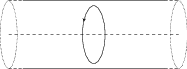# Ampere's Law

## Problems from IIT JEE

Problem (IIT JEE 1993): A current $I$ flows along the length of an infinitely long, straight, thin-walled pipe. Then the magnetic field

1. at all points inside the pipe is the same, but not zero.
2. at any point inside the pipe is zero.
3. is zero only on the axis of the pipe.
4. is different at different points inside the pipe.

Solution:By symmetry, the magnetic field inside the pipe is circumferential. Take a circular loop of radius $r$ with centre along the axis of the pipe. By symmetry, the magnitude of magnetic field is same throughout the loop. By Ampere's law, $\oint \vec{B}\cdot\mathrm{d}\vec{l}=B(2\pi r)=\mu_0I_\text{enc}$. Since $I_\text{enc}=0$, we get $B=0$ for all $r$. Thus, $B=0$ inside the pipe. The readers are encouraged to explain why there can not be a uniform field along the axis, similar to field of a solenoid.